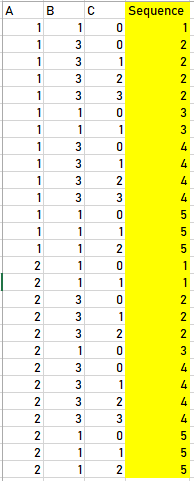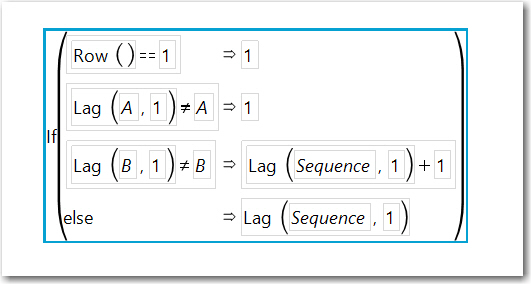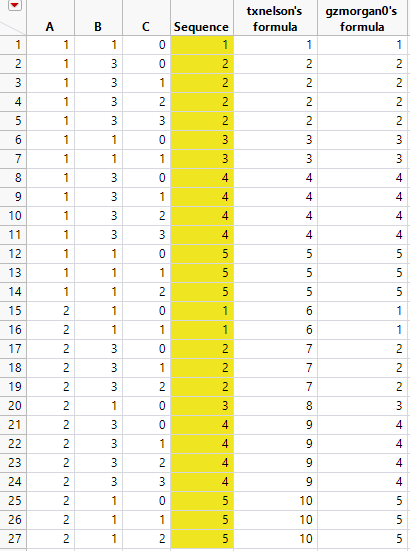Choose Language Hide Translation Bar
Highlighted

## Create sequential

I have data as below (column A, B, and C), I would like to create sequence column by add formula.1 ACCEPTED SOLUTION

Accepted Solutions
Highlighted

## Re: Create sequential

If you are trying to create the sequence column, here is the formula that recreates the column you showed. It is based upon the formula @txnelson  provided.

Attached is the table and the Sequence column with a formula.4 REPLIES 4
Highlighted

## Re: Create sequential

Here is one form of a formula that will work

``````if(row()==1, x=0);
If(:c==0, x=x+1);
x;``````
Jim
HighlightedJeff_PerkinsonCommunity Manager

## Re: Create sequential

The Sequence() function is what you're looking for.

-Jeff
Highlighted

## Re: Create sequential

If you are trying to create the sequence column, here is the formula that recreates the column you showed. It is based upon the formula @txnelson  provided.

Attached is the table and the Sequence column with a formula.Highlighted

## Re: Create sequential

Thanks all for solve my problem. I try all solution that you provide and gzmorgan0's formula is the best match my problem.

@gzmorgan0 wrote:

If you are trying to create the sequence column, here is the formula that recreates the column you showed. It is based upon the formula @txnelson  provided.

Attached is the table and the Sequence column with a formula.Article Labels

There are no labels assigned to this post.